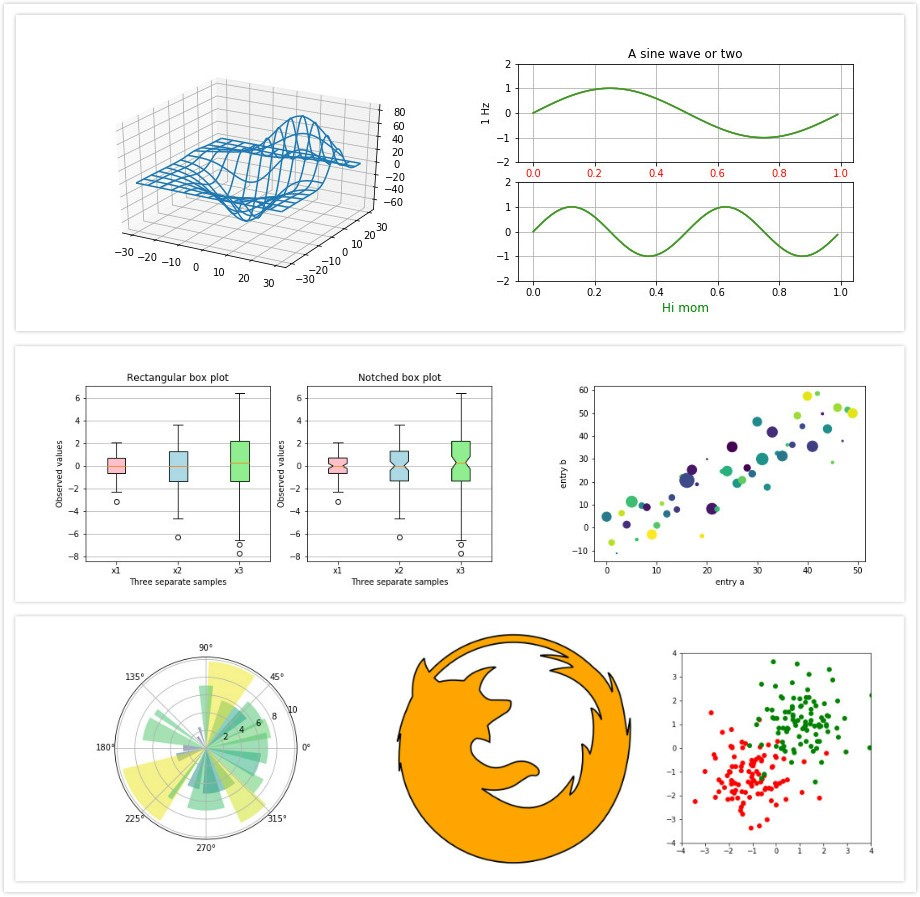##全国校区# 简便快捷的Python可视化库--matplotlib2020-07-02matplotlib 是一个用于创建二维图和图形的底层库，是python可视化库中规中矩的一种库，像大多数编程语言的标准库一样，读写、显示、子图、常见图形，通过matplotlib能够几行代码就能很轻松地画一些或简单或复杂地图形，线图、直方图、功率谱、条形图、错误图、散点图以及费笛卡尔坐标图等都不在话下。此外，matplotlib 还可以选择样式(style selection)，它模拟了像 ggplot2 和 xkcd 等很流行的美化工具,有许多流行的绘图库被设计为与matplotlib结合使用，已经成为python中公认的数据可视化工具。

matplotlib.pyplot.angle_spectrum 绘制电子波谱图
matplotlib.pyplot.bar 绘制柱状图
matplotlib.pyplot.barh 绘制直方图
matplotlib.pyplot.broken_barh 绘制水平直方图
matplotlib.pyplot.contour 绘制等高线图
matplotlib.pyplot.errorbar 绘制误差线
matplotlib.pyplot.hexbin 绘制六边形图案
matplotlib.pyplot.hist 绘制柱形图
matplotlib.pyplot.hist2d 绘制水平柱状图
matplotlib.pyplot.imshow 以图像显示
matplotlib.pyplot.pie 绘制饼状图
matplotlib.pyplot.quiver 绘制量场图
matplotlib.pyplot.scatter 散点图
matplotlib.pyplot.specgram 绘制光谱图
matplotlib.pyplot.subplot 绘制子图

OK